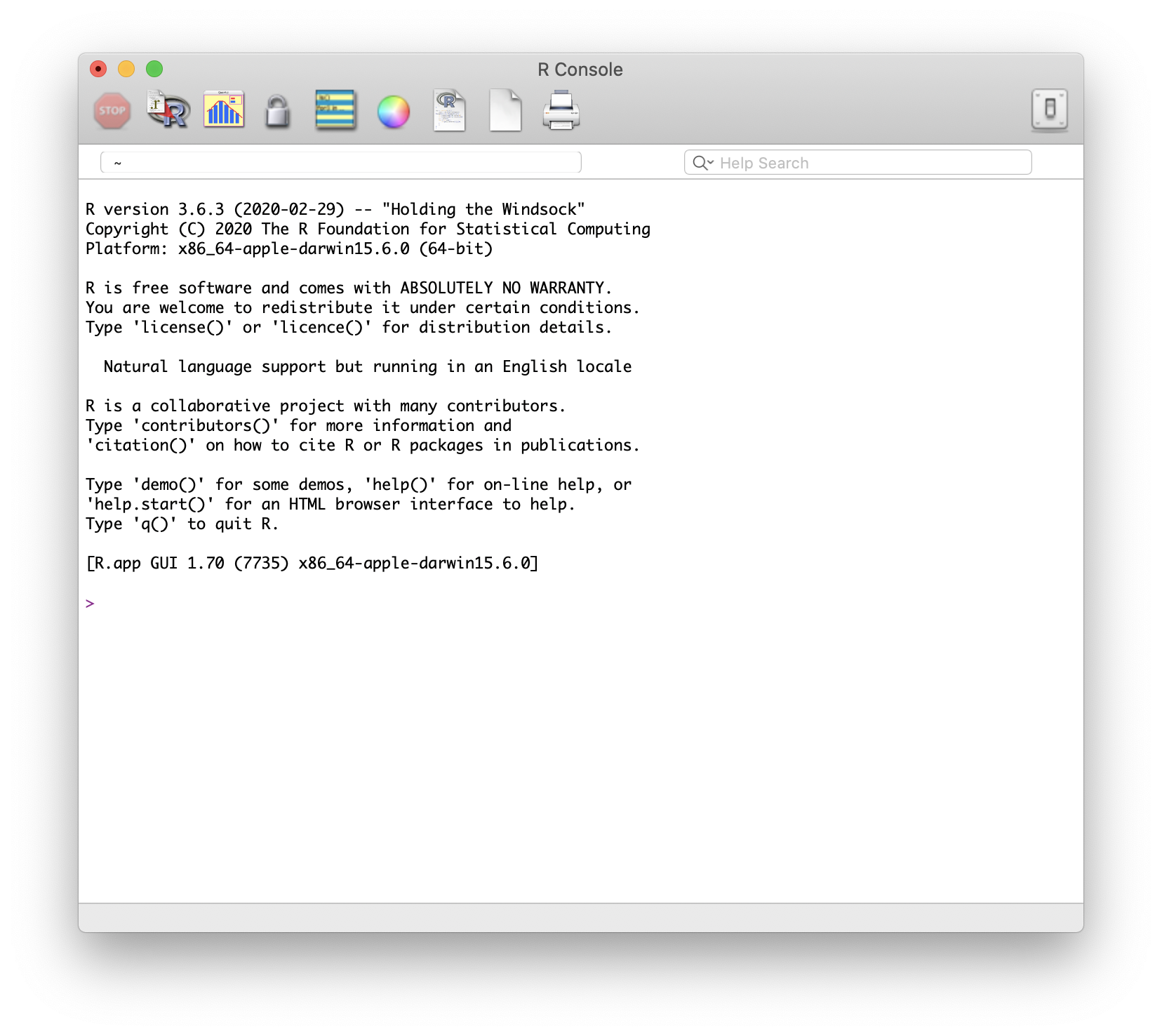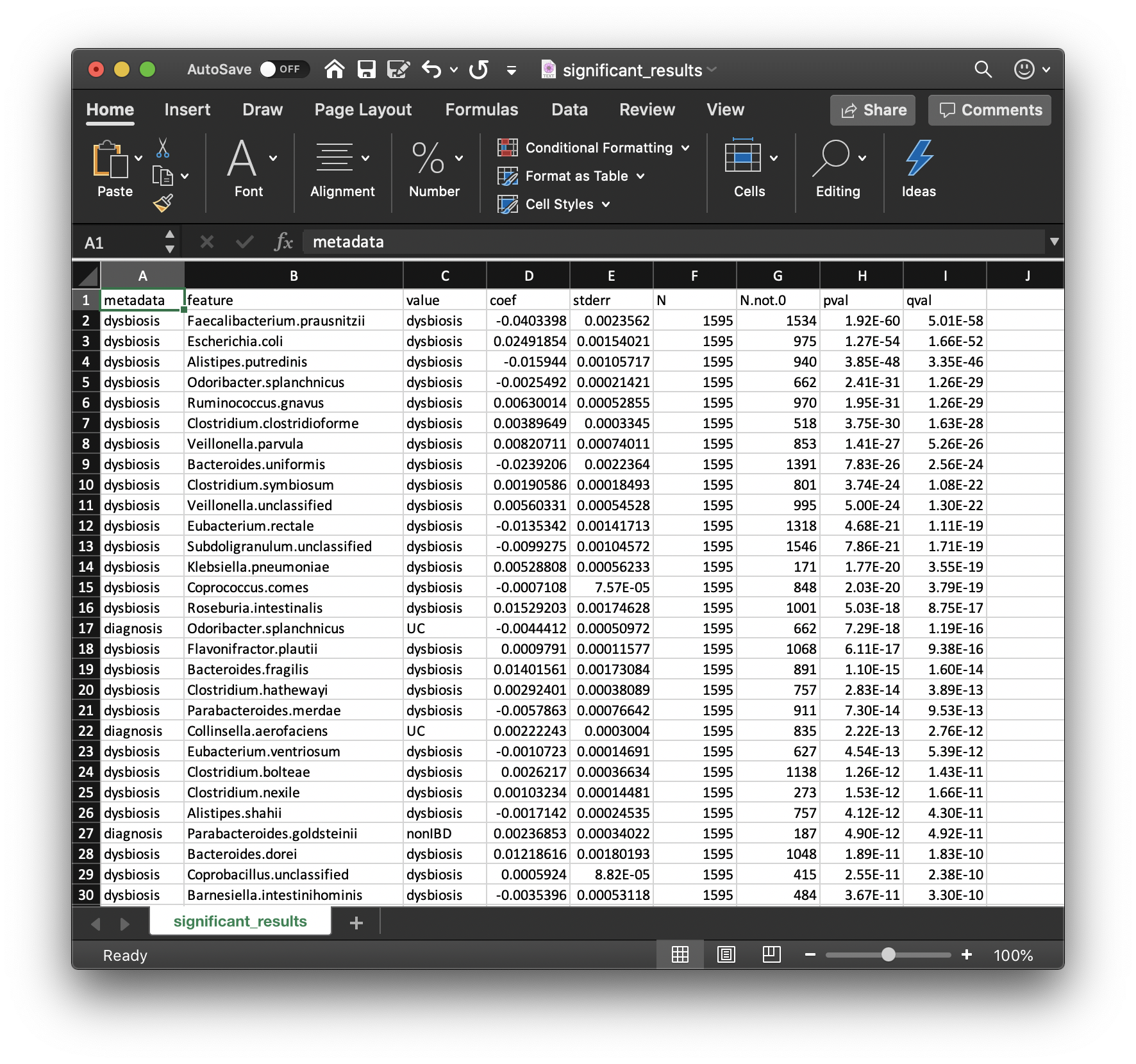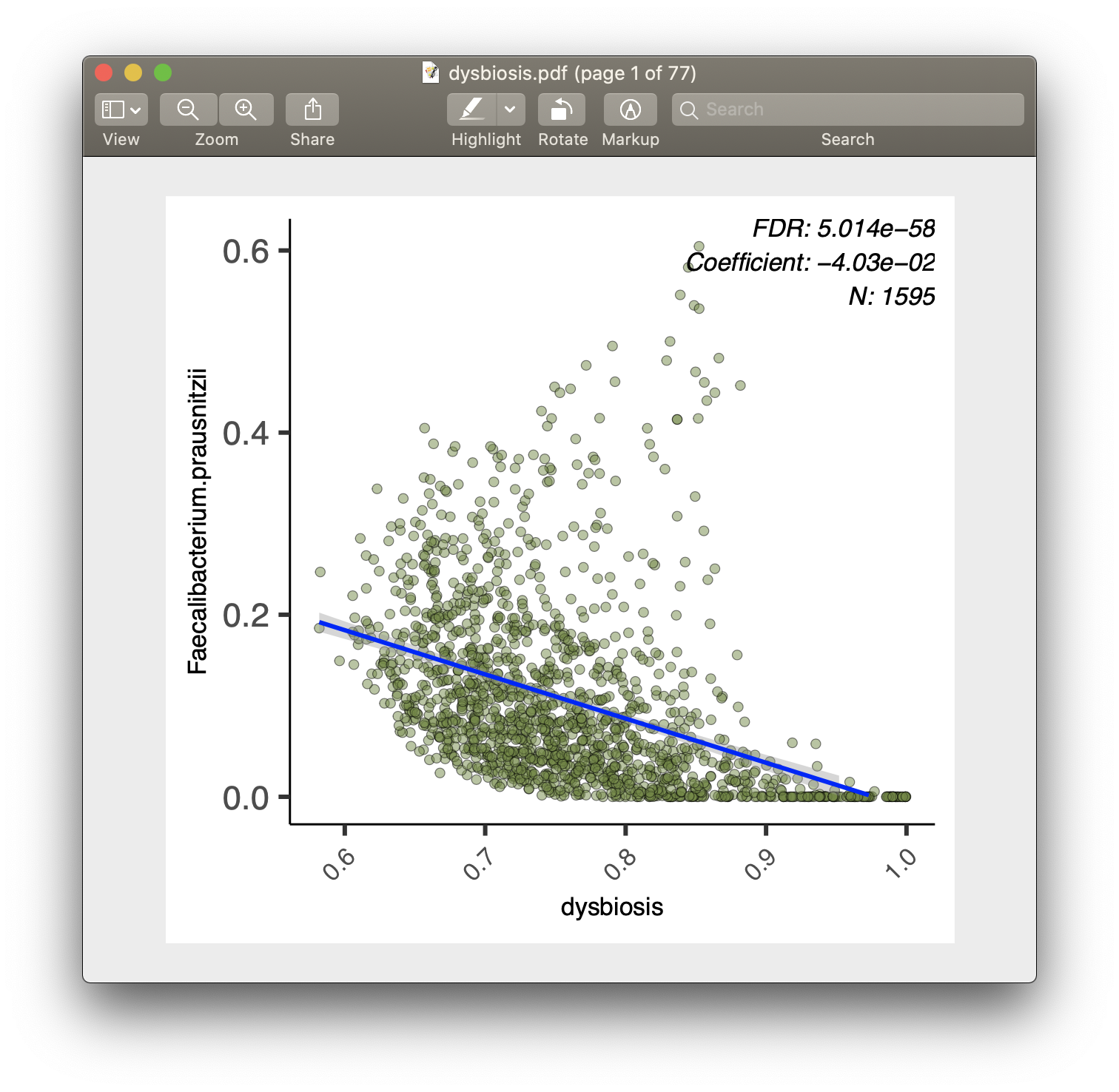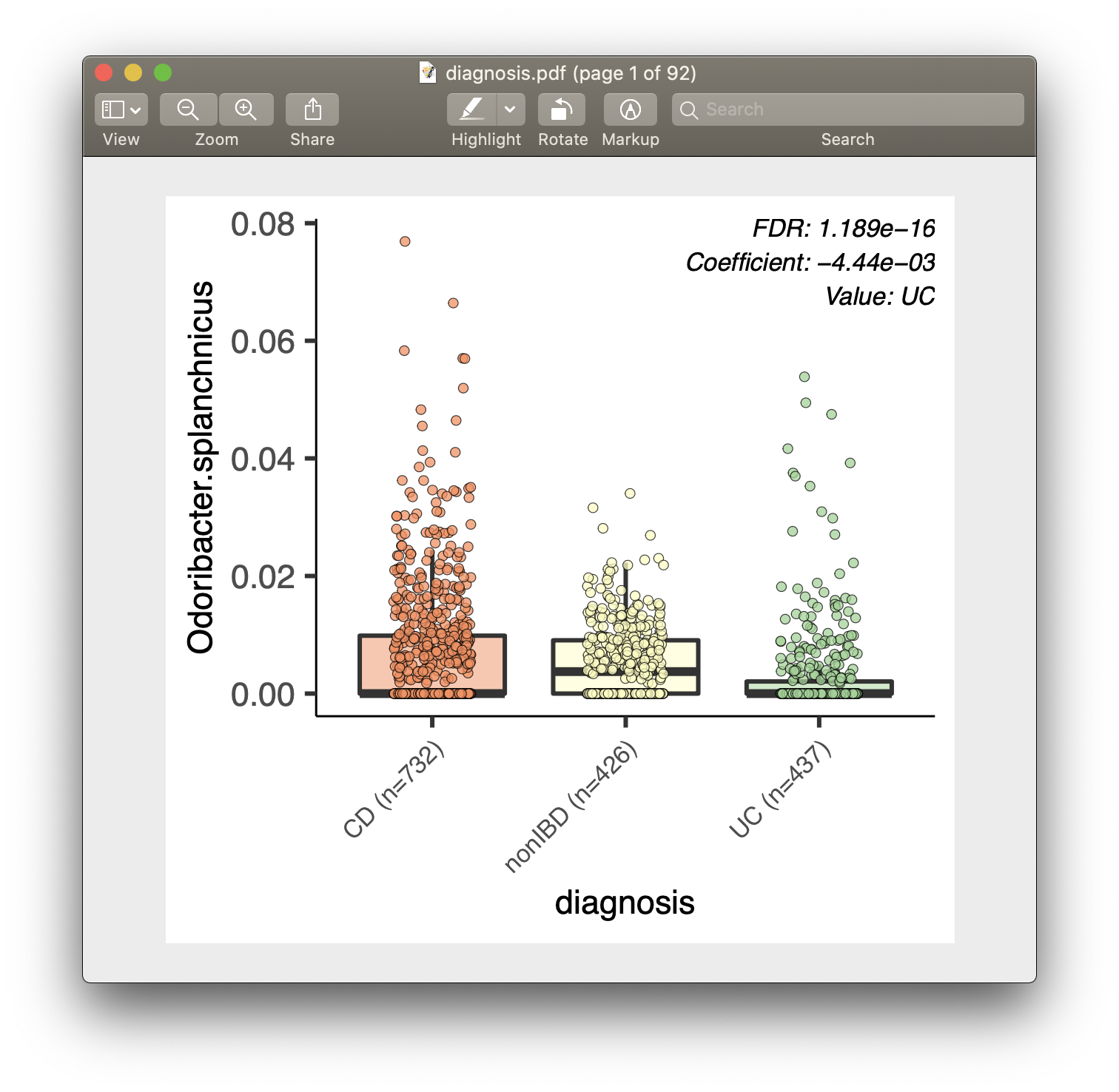{{ message }}
/ biobakery Public

Tutorials Home

# MaAsLin 2.0 tutorial

MaAsLin 2 is the next generation of MaAsLin (Microbiome Multivariable Association with Linear Models).

MaAsLin 2 is a comprehensive R package for efficiently determining multivariable association between phenotypes, environments, exposures, covariates and microbial meta-omics features. MaAsLin 2 relies on general linear models to accommodate most modern epidemiological study designs, including cross-sectional and longitudinal, along with a variety of data exploration, normalization, and transformation methods.

If you use the MaAsLin 2 software, please cite our manuscript:

Himel Mallick, Ali Rahnavard, Lauren J. McIver, Siyuan Ma, Yancong Zhang, Long H. Nguyen, Timothy L. Tickle, George Weingart, Boyu Ren, Emma Schwager, Suvo Catterjee, Kelsey N. Thompson, Jeremy E. Wilkinson, Ayshwarya Subramanian, Yiren Lu, Levi Waldron, Joseph N. Paulson, Eric A. Franzosa, Hector Corrada Bravo, Curtis Huttenhower. "Multivariable Association in Population-scale Meta-omics Studies". BioRxiv Preprint: https://doi.org/10.1101/2021.01.20.427420

## Support

If you have questions, please direct it to :
MaAsLin 2 Forum

## 1. Installing R

R is a programming language specializing in statistical computing and graphics. You can use R just the same as any other programming languages, but it is most useful for statistical analyses, with well-established packages for common tasks such as linear modeling, 'omic data analysis, machine learning, and visualization. We have created a short introduction tutorial here, but we note there are many other more comprehensive tutorials out there to learn R.

#### Installing R for the first time

You can download and install the free R software environment here. Note that you should download the latest release - this will ensure the R version you have is compatible with MaAsLin 2. Once you've finished the installation, locate the R software and launch it - you should have a window that looks like this:#### (Optional) the RStudio IDE

RStudio is a freely available IDE (integrated development environment) for R. It is a "wrapper" around R with some additional functionalities that makes programming in R a bit easier. Feel free to download RStudio and explore its interface - but it is not required for this tutorial.

#### Important: the correct R version

If you already have R installed, then it is possible that the R version you have does not support MaAsLin 2. The easiest way to check this is to launch R/RStudio, and in the console ("Console" pane in RStudio), type in the following command (without the `>` symbol):

`> sessionInfo()`

The first line of output message should indicate your current R version. For example:

```> sessionInfo()
R version 3.6.3 (2020-02-29)```

For MaAsLin 2 to install, you will need R >= 3.6.0. If your version is older than that, please refer to section Installing R for the first time to download the latest R. Note that RStudio users will need to have RStudio "switch" to this later version once it is installed. This should happen automatically for Windows and Mac OS users when you relaunch RStudio. For Linux users you might need to bind the correct R executable. For more information refer to here. Either way, once you have the correct version installed, launch the software and use `sessionInfo()` to make sure that you indeed have R >= 3.6.0.

## 2. Installing MaAsLin 2

### 2.1 With Bioconductor

Once you have the correct R version, you can install MaAsLin 2 with Bioconductor:

```if(!requireNamespace("BiocManager", quietly = TRUE))
install.packages("BiocManager")
BiocManager::install("Maaslin2")```

#### The `package ‘Maaslin2’ is not available` error

You might encounter an error message that states:

```Warning message:
package ‘Maaslin2’ is not available (for R version x.x.x) ```

This can be due to one of two reasons:

1. Your R version is too old. Check this with `sessionInfo()` - if it is older than 3.6, download the most updated version per instructions above.

2. You have an older version of Bioconductor. MaAsLin2 requires Bioconductor >= 3.10. You can check your Bioconductor version with `BiocManager::version()`. If the returned version is anything older than 3.10, you can update with

`BiocManager::install(version = "3.10")`

And then

`BiocManager::install("Maaslin2")`

### 2.2 With Docker

This is a place-holder right now.

## 3. Microbiome Association Detection with MaAsLin 2

### 3.1 MaAsLin 2 Input

Set up

Launch R and in the R script window:

```rm(list=ls())
getwd()
setwd("~/Desktop")
dir.create("R_Maaslin_tutorial") # Create a new directory
setwd("R_Maaslin_tutorial") # Change the current working directory
getwd() #check if directory has been successfully changed

#Load MaAsLin 2 package into the R environment
library(Maaslin2)
?Maaslin2```

MaAsLin 2 requires two input files, one for taxonomic or functional feature abundances, and one for sample metadata.

1. Data (or features) file
• This file is tab-delimited.
• Formatted with features as columns and samples as rows.
• The transpose of this format is also okay.
• Possible features in this file include microbes, genes, pathways, etc.
• This file is tab-delimited.
• Formatted with features as columns and samples as rows.
• The transpose of this format is also okay.

The data file can contain samples not included in the metadata file (along with the reverse case). For both cases, those samples not included in both files will be removed from the analysis. Also the samples do not need to be in the same order in the two files.

Example input files can be found in the `inst/extdata` folder of the MaAsLin 2 source. The files provided were generated from the HMP2 data which can be downloaded from https://ibdmdb.org/ .

```input_data = system.file("extdata", "HMP2_taxonomy.tsv", package="Maaslin2") # The abundance table file
input_data

#get the pathway (functional) data - place holder
```

`HMP2_taxonomy.tsv`: is a tab-demilited file with species as columns and samples as rows. It is a subset of the taxonomy file so it just includes the species abundances for all samples.

`HMP2_metadata.tsv`: is a tab-delimited file with samples as rows and metadata as columns. It is a subset of the metadata file so that it just includes some of the fields.

`pathabundance_relab.tsv`: is a tab-delimited file with samples as columns and pathway level features as rows. It is a subset of the pathway file so it just includes the species abundances for all samples.

Saving inputs as data frames

```df_input_data = read.table(file = input_data, header = TRUE, sep = "\t", row.names = 1, stringsAsFactors = FALSE)
df_input_data[1:5, 1:5]

df_input_path = data.frame(read.csv("./pathabundance_relab.tsv", sep = "\t", stringsAsFactors = F, row.names = 1))
df_input_path[1:5, 1:5]```

### 3.2 Running MaAsLin 2

Several different types of statistical models can be used for association testing in MaAsLin (e.g. simple linear, zero-inflated, count-based, etc.). We have evaluated their pros and cons in the associated manuscript, and while the default is generally appropriate for most analyses, a user might want to select a different model under some circumstances. This is true for many different microbial community data types (taxonomy or functional profiles), environments (human or otherwise), and measurements (counts or relative proportions), as long as alternative models are used with appropriately modified normalization/transformation schemes.

• For models, if your inputs are counts, then you can use `NEGBIN` and `ZINB`, whereas, for non-count (e.g. percentage, CPM, or relative abundance) input, you can use `LM` and `CPLM`.
• Among the normalization approaches implemented in MaAsLin 2, `TMM` and `CSS` only work on counts, and they also return normalized counts, unlike `TSS` and `CLR`. Therefore, if your inputs are counts, you can use the above two normalizations (i.e., `TMM`, `CSS`, or `NONE` (in case the data is already normalized)) without a further transformation (i.e. transform = `NONE`).
• Among the non-count models, `CPLM` requires the data to be positive. Therefore, any transformation that produces negative values will typically NOT work for `CPLM`.
• All the non-LM models use an intrinsic log link transformation due to their close connection to GLMs and they are recommended to be run with `transform = NONE`.
• Apart from that, `LM` is the only model that works on both positive and negative values (following normalization/transformation), and (as per our manuscript), it is generally much more robust to parameter changes (which are typically limited for non-LM models). Regarding whether to use `LM`, `CPLM`, or other models, intuitively, `CPLM` or a zero-inflated alternative should perform better in the presence of zeroes, but based on our benchmarking, we do not have evidence that `CPLM` is significantly better than `LM` in practice.
Model (-m ANALYSIS_METHOD) Data type Normalization (-n NORMALIZATION) Transformation (-t TRANSFORM)
LM count and non-count `TSS`,`CLR`, `NONE` `LOG`, `LOGIT`, `AST`,`NONE`
CPLM count and non-count `TSS`, `TMM`, `CSS`, `NONE` `NONE`
NEGBIN count `TMM`, `CSS`, `NONE` `NONE`
ZINB count `TMM`, `CSS`, `NONE` `NONE`

NOTE: generally you will also need to consider what thresholds you have used to filter the data (i.e. the prevalence and abundance threshold) to reduce outliers and false positives. See section 4.3.1 Prevalence and abundance filtering in MaAsLin 2 for some more details on this.

The following command runs MaAsLin 2 on the HMP2 data, running a multivariable regression model to test for the association between microbial species abundance versus IBD diagnosis and dysbiosis scores (`fixed_effects = c("diagnosis", "dysbiosis")`). For any categorical variable with more than 2 levels, you will also have to specify which variable should be the reference level by using (`reference = c("diagnosis,nonIBD")`). NOTE: adding a space between the variable and level might result in the wrong reference level being used. Output are generated in a folder called `demo_output` under the current working directory (`output = "demo_output"`). In this case, the example input data has been pre-normalized and pre-filtered, so we turn off the default normalization and prevalence filtering as well.

```fit_data = Maaslin2(
input_data = input_data,
min_prevalence = 0,
normalization = "NONE",
output = "demo_output",
fixed_effects = c("diagnosis", "dysbiosis"),
reference = c("diagnosis,nonIBD"))```

#### Providing data frames as input

If you are familiar with the data frame class in R, you can also provide data frames for `input_data` and `input_metadata` for MaAsLin 2, instead of file names. One potential benefit of this approach is that it allows you to easily manipulate these input data within the R environment.

```fit_data2 = Maaslin2(
input_data = df_input_data,
min_prevalence = 0,
normalization = "NONE",
output = "demo_output2",
fixed_effects = c("diagnosis", "dysbiosis"),
reference = c("diagnosis,nonIBD"))```
• Question: how would I run the same analysis, but only on CD and nonIBD subjects?
• Hint: try `?dplyr::filter()`

### 3.3 MaAsLin 2 Output

#### Significant associations

Perhaps the most important output from MaAsLin 2 is the list of significant associations. These are provided in `significant_results.tsv`:These are the full list of associations that pass MaAsLin 2's significance threshold, ordered by increasing q-values. Columns are:

• `metadata`: the variable name being associated with a microbial feature.

• `feature`: the microbial feature (taxon, gene, pathway, etc.).

• `value`: for categorical features, the specific feature level for which the coefficient and significance of association is being reported.

• `coef`: the model coefficient value (effect size).

• Coefficients for categorical variables indicate the contrast between the category specified in `value` versus the reference category.
• `stderr`: the standard error from the model.

• `N`: the total number of samples used in the model for this association (since e.g. missing values can be excluded).

• `N.not.0`: the total of number of these samples in which the feature is non-zero.

• `pval`: the nominal significance of this association.

• `qval`: the corrected significance is computed with `p.adjust` with the correction method (BH, etc.).

• Question: how would you interpret the first row of this table?

For each of the significant associations in `significant_results.tsv`, MaAsLin 2 also generates visualizations for inspection (boxplots for categorical variables, scatter plots for continuous). These are named as "metadata_name.pdf". For example, from our analysis run, we have the visualization files `dysbiosis.pdf` and `diagnosis.pdf`:#### Full list of output files

MaAsLin 2 generates two types of output files: data and visualization.

1. Data output files
• `significant_results.tsv`
• As introduced above.
• `all_results.tsv`
• Same format as `significant_results.tsv`, but include all association results (instead of just the significant ones).
• You can also access this table within R using `fit_data\$results`.
• `residuals.rds`
• This file contains a data frame with residuals for each feature.
• `maaslin2.log`
• It includes all settings, warnings, errors, and steps run.
2. Visualization output files
• `heatmap.pdf`
• This file contains a heatmap of the significant associations.
• `[a-z/0-9]+.pdf`
• A plot is generated for each significant association.
• Scatter plots are used for continuous metadata.
• Box plots are for categorical data.
• Data points plotted are after normalization, filtering, and transform.

### 3.4 MaAsLin 2 on functional profiles

If your functional tables have been produced by HUMAnN and been normalized with the `humann_renorm_table` script, then you don’t need to perform any additional normalization – the effects of sequencing depth will have already been removed (which is what we will be using in this tutorial). We tend to work with CPM units because we find them to be more convenient, but they are numerically equivalent to relative abundances for modeling purposes (CPM = RA * 1e6).

Otherwise - you will want to use the same thoughts above about model/normalization/transformation as above.

Some other quick tips for using MaAsLin 2 on functional profiles:

• To reduce the number of features, you typically want to run either the unstratified functional features (or a filtered subset of the stratified features)
• You may also want to remove functional features that are highly correlated to one specific taxon (i.e. likely contributed by that microbe), since these can be better-explained by taxonomic changes.
```#This can also be done with with the HUMAnN 3 untiliy `humann_split_stratified_table`
unstrat_pathways <-function(dat_path){
temp = dat_path[!grepl("\\|",rownames(dat_path)),]
return(temp)
}

df_input_path = unstrat_pathways(df_input_path)```

Run the same model as above on the MetaCyc pathway table generated from the bioBakery workflows.

```fit_func = Maaslin2(
input_data = df_input_path,
output = "demo_functional",
fixed_effects = c("diagnosis", "dysbiosis"),
reference = c("diagnosis,nonIBD"),
min_abundance = 0.0001,
min_prevalence = 0.1)```

Note: Here we have the samples in the columns and the features in the rows and MaAsLin 2 correctly identified this and was able to match the samples.

We have also recently published on using MaAsLin 2 to analyze differential features in metatranscriptomic data (MTX), for further information on that please see our recent publication.

Some helpful tips and tricks (HUMAnN related)

Q: Does the output of the functional run make sense? What are some of the important features to investigate further?

### 4.1 Interactions

Many statistical analysis are interested in testing for interaction between certain variables. Unfortunately, MaAsLin 2 does not yet provide a direct interface for this. Instead, the user needs to create artificial interaction columns as additional `fixed_effects` terms. Using the above fit as an example, to test for the interaction between `diagnosis` and `dysbiosis`, I can create two additional columns: `CD_dysbiosis` and `UC_dysbiosis` (since the reference for `diagnosis` is `nonIBD`):

```df_input_metadata\$CD_dysbiosis = (df_input_metadata\$diagnosis == "CD") *
fit_data_interaction = Maaslin2(
input_data = df_input_data,
min_prevalence = 0,
normalization = "NONE",
output = "demo_output_interaction",
fixed_effects = c("diagnosis", "dysbiosis", "CD_dysbiosis", "UC_dysbiosis"),
reference = c("diagnosis,nonIBD"))```

### 4.2 Random Effects

Certain studies have a natural "grouping" of sample observations, such as by subject in longitudinal designs or by family in family designs. It is important for statistic analysis to address the non-independence between samples belonging to the same group MaAsLin 2 provides a simple interface for this through the parameter `random_effects`, where the user can specify the grouping variable to run a mixed effect model instead. For example, we note that HMP2 is a longitudinal design where the same subject (column `subject`) can have multiple samples. We thus ask MaAsLin 2 to use subject as its random effect grouping variable:

```fit_data_random = Maaslin2(
input_data = df_input_data,
min_prevalence = 0,
normalization = "NONE",
output = "demo_output_random",
fixed_effects = c("diagnosis", "dysbiosis"),
random_effects = c("subject"),
reference = c("diagnosis,nonIBD"))```

If you are interested in testing the effect of time in a longitudinal study, then the time point variable should be included in `fixed_effects` during your MaAsLin 2 call.

• Question: intuitively, can you think of a reason why it is important to address non-independence between samples?
• Hint: imagine the simple scenario where you have two subject, one case and one control, each has two samples.
• What is the effective sample size, when samples of the same subject are highly independent, versus when they are highly correlated?

MaAsLin 2 provide many parameter options for different data pre-processing (normalization, filtering, transfomation) and other tasks. The full list of these options are:

• `min_abundance`
• The minimum abundance for each feature [ Default: `0` ]
• `min_prevalence`
• The minimum percent of samples for which a feature is detected at minimum abundance [ Default: `0.1` ]
• `max_significance`
• The q-value threshold for significance [ Default: `0.25` ]
• `normalization`
• The normalization method to apply [ Default: `"TSS"` ] [ Choices: `"TSS"`, `"CLR"`, `"CSS"`, `"NONE"`, `"TMM"` ]
• `transform`
• The transform to apply [ Default: `"LOG"` ] [ Choices: `"LOG"`, `"LOGIT"`, `"AST"`, `"NONE"` ]
• `analysis_method`
• The analysis method to apply [ Default: `"LM"` ] [ Choices: `"LM"`, `"CPLM"`, `"ZICP"`, `"NEGBIN"`, `"ZINB"` ]
• `correction`
• The correction method for computing the q-value [ Default: `"BH"` ]
• `standardize`
• Apply z-score so continuous metadata are on the same scale [ Default: `TRUE` ]
• `plot_heatmap`
• Generate a heatmap for the significant associations [ Default: `TRUE` ]
• `heatmap_first_n`
• In heatmap, plot top N features with significant associations [ Default: `50` ]
• `plot_scatter`
• Generate scatter plots for the significant associations [ Default: `TRUE` ]
• `cores`
• The number of R processes to run in parallel [ Default: `1` ]
• `reference`
• The factor to use as a reference for a variable with more than two levels provided as a string of 'variable,reference' semi-colon delimited for multiple variables.

#### 4.3.1 Prevalence and abundance filtering in MaAsLin 2

Typically, it only makes sense to test for feature-metadata associations if a feature is non-zero "enough" of the time. "Enough" can vary between studies, but a 10-50% minimum prevalence threshold is not unusual (and up to 70-90% can be reasonable). Since we're already using a small, selected subset of demo data, we'll use `min_prevalence = 0.1` to test only features with at least 10% non-zero values. Note: this is the default parameter setting in MaAsLin 2.

Similarly, it's often desirable to test only features that reach a minimum abundance threshold in at least this many samples. By default, MaAsLin 2 will consider any non-zero value to be reliable, and if you've already done sufficient QC in your dataset, this is appropriate. However, if you'd like to filter more or be conservative, you can set a minimum abundance threshold like `min_abundance = 0.0001` to test only features reaching at least this (relative) abundance.

Putting these arguments together, we can run MaAsLin 2 (and it'll go even faster!) using both:

```fit_data_filter = Maaslin2(
input_data = df_input_data,
normalization = "NONE",
output = "demo_output_filter",
fixed_effects = c("diagnosis", "dysbiosis"),
reference = c("diagnosis,nonIBD"),
random_effects = c("subject"),
min_prevalence = 0.1,
min_abundance = 0.0001)```

## 5. Command Line Interface

MaAsLin 2 can also be run with a command line interface. For example, the demo analysis can be performed with:

``````Maaslin2.R --transform=AST --fixed_effects="diagnosis,dysbiosis"  inst/extdata/HMP2_taxonomy.tsv inst/extdata/HMP2_metadata.tsv demo_output --reference="diagnosis,nonIBD"
``````
• Make sure to provide the full path to the MaAsLin 2 executable (ie ./R/Maaslin2.R).
• In the demo command:
• `HMP2_taxonomy.tsv` is the path to your data (or features) file
• `HMP2_metadata.tsv` is the path to your metadata file
• `demo_output` is the path to the folder to write the output

Full help documentation:

``````\$ Maaslin2.R --help
Usage: ./R/Maaslin2.R [options] <data.tsv> <metadata.tsv> <output_folder>

Options:
-h, --help
Show this help message and exit

-a MIN_ABUNDANCE, --min_abundance=MIN_ABUNDANCE
The minimum abundance for each feature [ Default: 0 ]

-p MIN_PREVALENCE, --min_prevalence=MIN_PREVALENCE
The minimum percent of samples for which a feature
is detected at minimum abundance [ Default: 0.1 ]

-s MAX_SIGNIFICANCE, --max_significance=MAX_SIGNIFICANCE
The q-value threshold for significance [ Default: 0.25 ]

-n NORMALIZATION, --normalization=NORMALIZATION
The normalization method to apply [ Default: TSS ]
[ Choices: TSS, CLR, CSS, NONE, TMM ]

-t TRANSFORM, --transform=TRANSFORM
The transform to apply [ Default: LOG ]
[ Choices: LOG, LOGIT, AST, NONE ]

-m ANALYSIS_METHOD, --analysis_method=ANALYSIS_METHOD
The analysis method to apply [ Default: LM ]
[ Choices: LM, CPLM, NEGBIN, ZINB ]

-r RANDOM_EFFECTS, --random_effects=RANDOM_EFFECTS
The random effects for the model, comma-delimited
for multiple effects [ Default: none ]

-f FIXED_EFFECTS, --fixed_effects=FIXED_EFFECTS
The fixed effects for the model, comma-delimited
for multiple effects [ Default: all ]

-c CORRECTION, --correction=CORRECTION
The correction method for computing the
q-value [ Default: BH ]

-z STANDARDIZE, --standardize=STANDARDIZE
Apply z-score so continuous metadata are
on the same scale [ Default: TRUE ]

-l PLOT_HEATMAP, --plot_heatmap=PLOT_HEATMAP
Generate a heatmap for the significant
associations [ Default: TRUE ]

-i HEATMAP_FIRST_N, --heatmap_first_n=HEATMAP_FIRST_N
In heatmap, plot top N features with significant
associations [ Default: TRUE ]

-o PLOT_SCATTER, --plot_scatter=PLOT_SCATTER
Generate scatter plots for the significant
associations [ Default: TRUE ]

-e CORES, --cores=CORES
The number of R processes to run in parallel
[ Default: 1 ]

-r REFERENCE, --reference='VARIABLE,REFERENCE'
The factor to use as a reference for a
variable with more than two levels
provided as a string of 'variable,reference'
semi-colon delimited for multiple variables.
``````